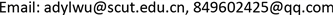1华南理工大学经济与贸易学院电子商务系，广东 广州

2华南理工大学现代服务业研究院商务智能研究中心，广东 广州1. 引言

2. 研究现状

3. 新的热点话题发现方法与建模的理论基础3.1. 考虑时变因素的话题热度(人气度)的计算

I n f b , u ( t ) = − d ( t − t 0 ) 2 + | u followed | H T = ∑ Δ I n f T = ∑ ( ∑ i = 1 n I n f b i ) (1)

3.2. 词语动能

“词语动能”(Word Kinetic Energy, WKE)的定义：词语因为运动而具有的能量，称为“词语动能”。在微博话题传播的情境中，“词语运动”是指词语随着文本数量增长而增长的这样一个动态过程，根据词语的增长速度计算而得到的一种值，即“词语动能”。词语的动能，是网络词语的突发性大小的一种度量，用词语的平均增长速度来进行计算 。词语动能的物理意义表示词语所具有的能量，是一种对词语突发性的衡量；而词语动能的社会意义，则表现为话题传播影响力的强度。

E = ( 1 / 2 ) m ν 2 (2)

v k , j = { 0 , k = 0 m k , j − m k − 1 , j T k − 1 , k , k ≠ 0 (3)

T k − 1 , k = T k − m i d d l e − T k − 1 − m i d d l e (4)

E k , j = α ⋅ t f k j ⋅ v k , j 2 (5)

4. 问题描述与模型构建4.1. 问题定义

1) 研究问题一：如何基于传播周期对词语突发性进行衡量与评估？

2) 研究问题二：如何基于传播周期对话题热度(人气度)进行时效性考量？

4.2. 基于词语动能的文本聚类模型

1) 对经典特征词权重计算方法TF-IDF的改进

ω i j = t f i j ⋅ log ( N / n j + 0.01 ) ∑ p = 1 k [ t f i j ⋅ log ( N / n j + 0.01 ) ] 2 + E k , j (6)

2) 引入词语动能的文本相似度的计算

cos ( x , y ) = ∑ k = 1 n ω x k × ω y k ∑ k = 1 n ω x k 2 × ∑ k = 1 n ω y k 2 (7)

4.3. 优化算法设计

4.4. 基于传播周期的话题热度(人气度)计算

T H i = ∑ k = 0 n b h i k − β ( t − t 0 ) 2 = ∑ k = 0 n ( 2 r e k + c m k + f l k ) − β ( t − t o ) 2 (8)

5. 实验及结果分析

5.1. 实验语料准备

5.2. 模型和算法性能评测

TDT会议对话题检测制定了一种规范的评价标准，评测指标主要有：召回率、准确率、漏检率、错检率、F值以及误测开销 。该评价标准同样适用于微博平台的话题检测。本文选用话题检测常用的评价指标即召回率、错检率来对模型与算法的性能进行测试。

R = D / T ∗ 100 % (9)

P F A = F A / N T ∗ 100 % (10)

5.3. 实验结果5.3.1. 动能权重系数 α 的取值

5.3.2. 实验结果及算法评测

Experimental results of topic discovery algorith

Comparison of experimental result

1《西游记》导演杨洁去世自杀袭击者引诱儿童《西游记》导演杨洁去世
2叙利亚自杀袭击者用薯片引诱儿童独生女留学嫁老外不归天舟一号在海南文昌发射
3一个在爆炸袭击中幸存的叙利亚小女孩的微笑白百何出轨事件拔河比赛只剩一人苦撑
4小学拔河比赛只剩他一人苦撑，决不放弃天舟一号发射自杀袭击者用薯片引诱儿童

Topic recall rate obtained by two algorithm

1《西游记》导演杨洁去世80.8286.35
2叙利亚自杀袭击者用薯片引诱儿童81.9885.21
3一个在爆炸袭击中幸存的叙利亚小女孩的微笑80.2187.31
4小学拔河比赛只剩他一人苦撑，决不放弃81.2485.24

Topic error detection rate obtained by two algorithm

1《西游记》导演杨洁去世0.810.67
2叙利亚自杀袭击者用薯片引诱儿童0.790.65
3一个在爆炸袭击中幸存的叙利亚小女孩的微笑0.820.72
4小学拔河比赛只剩他一人苦撑，决不放弃0.830.61

6. 结论